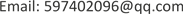1. 引言

2. 研究背景及现状2.1. 目标检测算法

2.2. 超分辨率算法

3. 基于目标检测网络的超分辨率重建模型

X为原图， X ′ 为X的退化图像，Y为X中用户感兴趣的区域，x为 中用户感兴趣的区域，y为生成网络生成的图像。T为目标检测网络，用于获取X中的(x, y, h, w, confidence)信息，G为图像生成网络，D为鉴别网络。

3.1. 目标检测卷积神经网络结构设计

l o s s = λ c o o r d ∑ i = 0 l . w ∗ l . h ∑ j = 0 l . n 1 i j o b j [ ( x i − x ^ i ) 2 + ( y i − y ^ i ) 2 ]     + λ c o o r d ∑ i = 0 l . w ∗ l . h ∑ j = 0 l . n 1 i j o b j [ ( w i − w ^ i ) 2 + ( h i − h ^ i ) 2 ]     + λ n o o b j ∑ i = 0 l . h ∗ l . w ∑ j = 0 l . n 1 i j n o o b j ( C i − C ^ i ) 2 + λ o b j ∑ i = 0 l . h ∗ l . w ∑ j = 0 l . n 1 i j o b j ( C i − C ^ i ) 2

3.2. 图像生成卷积神经网络结构设计

3.3. 图像判别卷积神经网络结构设计

3.4. 超分辨网络的目标函数

L c G A N ( G , D ) = E x , y [ log D ( x , y ) ] + E x , z [ log ( 1 − D ( x , G ( x , z ) ) ) ]

L L 1 ( G ) = E x , y , z [ ‖ y − G ( x , z ) ‖ 1 ]

G = arg min G max D L c G A N ( G , D ) + λ L L 1 (G)

4. 实验结果与分析

Test results on datasets using different super-resolution method

Yolo2pix×424.5490.79177.8
discogan23.4010.72413.2
pix2pix23.8910.73112.9
pocs18.9480.6312034
bilinear23.2660.7528.8
bicubic23.8640.77713.6
Yolo2pix×922.7910.71578.2
discogan22.0310.64713.3
pix2pix22.1540.65213.1
pocs17.5490.5812154
bilinear21.8150.6828.8
bicubic22.1480.69914.8
Yolo2pix×1621.7460.67578.3
discogan21.0500.62713.3
pix2pix21.0470.63613.2
pocs16.7580.5322253
bilinear20.9580.6299.6
bicubic21.2410.64515.2

5. 结语